1. Worksheets>
2. Math>
3. Geometry>
4. Ordered Pairs

# Ordered Pairs and Coordinate Plane Worksheets

The worksheets in this page cover identifying quadrants, axes, identifying ordered pairs, coordinates, plotting points on coordinate plane and other fun worksheets to reinforce the knowledge in ordered pairs. Also contains mystery pictures, moving points using position and direction, identifying shapes and more.

## Ordered Pairs and Plotting Points

Identifying Position: Real-life Objects

Identify the position of each item placed on the coordinate grid. Also find the item located at given ordered pairs.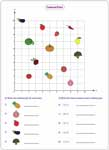Only Positive:

Identifying Ordered Pairs

There are two sections in each worksheet. First section is about identifying points on the coordinate plane. Second section is about writing ordered pair for each given point.Only Positive:

Plotting Points

Plot the points on the first coordinate grid and draw shapes for each ordered pair on the second coordinate grid.Only Positive:

Ordered Pairs and Plotting Points: Mixed

Each worksheet contains 21 problems finding the coordinates and plotting points on the grid.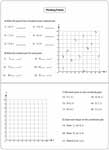Only Positive:

These are the basic worksheets in learning quadrants. Identify the quadrant belongs to each object and vice versa.There are some points on the coordinate grid. Tell the points, belong to each quadrant or axis.Look at the sign of the coordinates to identify the quadrant. If x-coordinate is zero, then the point is on the y-axis. If y-coordinate is zero, then the point is on the x-axis.## Fun with Ordered Pairs

Showing the Route

Help to reach the destination. Show the route by plotting the ordered pairs and connecting them with the lines.Only Positive:

Identifying Shapes

Identify the shape by plotting and joining the points in the given order. Do not forget to join the end points.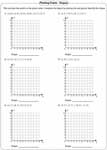Only Positive:

Length of the Line Segment

Draw line segments by plotting and joining the points. Additionally, find the length of the line segments.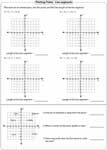Mystery Picture

Plot the points and join them as per the direction. Identify the mystery picture. Both single sequence and multiple sequences are available.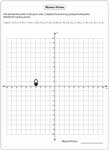Single Sequence:

Multiple Sequences:

## Changing Position or Direction

Moving the Points

Start at one point, follow the direction and write the ordered pair for the end point. There are two types, position and direction. Position is based on up, down, right and up. Direction is based on north, east, west and south.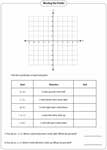Based on Positions:

Based on Directions:

Moving Around the Points

Look around the points and answer the questions. Different theme is used in each worksheet.The whole batch of worksheets on ordered pairs and coordinate plane can be downloaded instantly.

Related Worksheets